# FRDM-STBC-AGM01 - Bare metal example project

cancel
Showing results for
Did you mean:

# FRDM-STBC-AGM01 - Bare metal example project

## FRDM-STBC-AGM01 - Bare metal example project

Hi Everyone,

In this tutorial I intend to run through my simple bare metal example code I created for the Freescale FRDM-KL25Z platform and the FRDM-STBC-AGM01 board containing a three axis accelerometer + magnetometer (FXOS8700CQ) and a three axis gyroscope (FXAS21002C). I will not cover the Sensor Fusion library and the ISF which also support this board. The FreeMASTER tool is used to visualize all the data that are read from both sensors using an interrupt technique through the I2C interface.

This example illustrates:

1. Initialization of the MKL25Z128 MCU (mainly I2C and PORT modules).

2. I2C data write and read operations.

3. Initialization of the FXOS8700CQ and FXAS21002C.

4. Simple accelerometer offset calibration based on the AN4069.

5. Output data reading using an interrupt technique.

6. Conversion of the output raw values to real values in g’s, µT, dps and °C.

7. Visualization of the calculated values in the FreeMASTER tool.

1. As you can see in the FRDM-STBC-AGM01 schematic, both sensors are controlled via I2C by default. With jumpers J6 and J7 in their default position (2-3), the I2C signals are routed to the I2C1 module (PTC1 and PTC2 pins) of the KL25Z MCU. The INT1_8700 output is connected to the PTD4 pin and the INT1_21002 pin to the PTA5 pin of the KL25Z MCU. These both interrupt pins are configured as push-pull active-low outputs, so the corresponding PTD4/PTA5 pin configuration is GPIO with an interrupt on falling edge.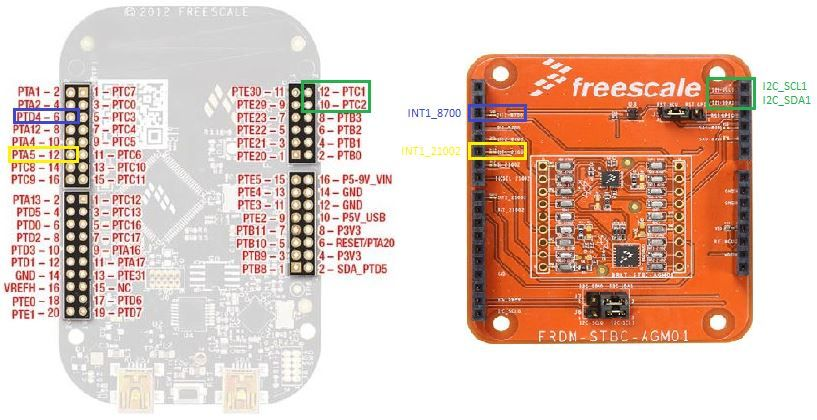The MCU is, therefore, configured as follows.

void MCU_Init(void)

{

//I2C1 module initialization

SIM_SCGC4 |= SIM_SCGC4_I2C1_MASK;        // Turn on clock to I2C1 module

SIM_SCGC5 |= SIM_SCGC5_PORTC_MASK;       // Turn on clock to Port C module

PORTC_PCR1 |= PORT_PCR_MUX(0x2);         // PTC1 pin is I2C1 SCL line

PORTC_PCR2 |= PORT_PCR_MUX(0x2);         // PTC2 pin is I2C1 SDA line

I2C1_F  |= I2C_F_ICR(0x14);              // SDA hold time = 2.125us, SCL start hold time = 4.25us, SCL stop hold time = 5.125us

I2C1_C1 |= I2C_C1_IICEN_MASK;            // Enable I2C1 module

//Configure the PTD4 pin (connected to the INT1 of the FXOS8700CQ) for falling edge interrupts

SIM_SCGC5 |= SIM_SCGC5_PORTD_MASK;       // Turn on clock to Port D module

PORTD_PCR4 |= (0|PORT_PCR_ISF_MASK|      // Clear the interrupt flag

PORT_PCR_MUX(0x1)|      // PTD4 is configured as GPIO

PORT_PCR_IRQC(0xA));    // PTD4 is configured for falling edge interrupts

//Configure the PTA5 pin (connected to the INT1 of the FXAS21002) for falling edge interrupts

SIM_SCGC5 |= SIM_SCGC5_PORTA_MASK;       // Turn on clock to Port A module

PORTA_PCR5 |= (0|PORT_PCR_ISF_MASK|      // Clear the interrupt flag

PORT_PCR_MUX(0x1)|      // PTA5 is configured as GPIO

PORT_PCR_IRQC(0xA));    // PTA5 is configured for falling edge interrupts

//Enable PORTD interrupt on NVIC

NVIC_ICPR |= 1 << ((INT_PORTD - 16)%32);

NVIC_ISER |= 1 << ((INT_PORTD - 16)%32);

//Enable PORTA interrupt on NVIC

NVIC_ICPR |= 1 << ((INT_PORTA - 16)%32);

NVIC_ISER |= 1 << ((INT_PORTA - 16)%32);

}

2. The 7-bit I2C slave address of the FXOS8700CQ is 0x1E since both SA0 and SA1 pins are shorted to GND. The address of the FXAS21002C is 0x20 since SA0 pin is also shorted to GND.

The two screenshots below show the write operation which writes the value 0x25 to the CTRL_REG1 (0x2A) of the FXOS8700CQ and 0x16 to the CTRL_REG1 (0x13) of the FXAS21002C.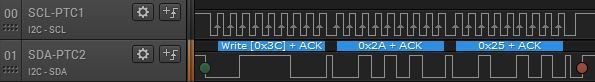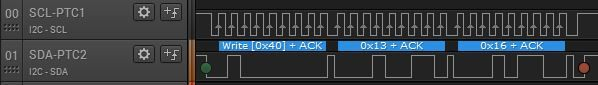Here is the single byte read from the WHO_AM_I register. As you can see, it returns the correct value 0xC7 for the FXOS8700CQ and 0xD7 for the FXAS21002C.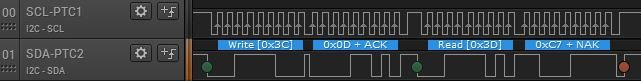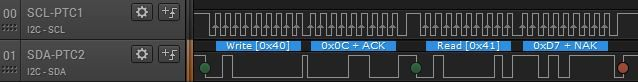Finally, a burst read of 12 bytes from the FXOS8700CQ output data registers (0x01 – 0x06 and 0x33 – 0x38) and 6 bytes from the FXAS21002C output data registers (0x01 – 0x06) is shown below.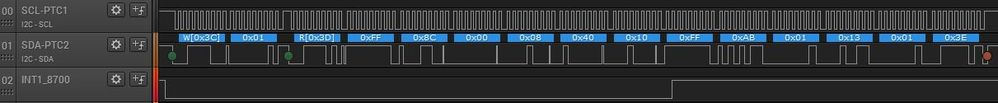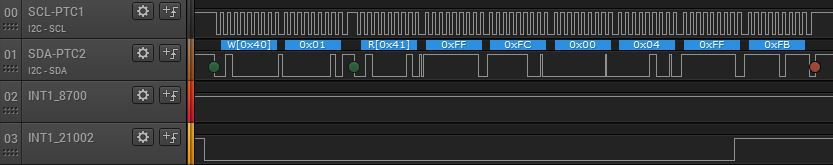3. At the beginning of the initialization, all registers are reset to their default values by setting the RST bit of the CTRL_REG2 register. Then the FXOS8700CQ is initialized as shown below.

void FXOS8700CQ_Init (void)

{

I2C_WriteRegister(FXOS8700CQ_I2C_ADDRESS, CTRL_REG2, 0x40);          // Reset all registers to POR values

Pause(0x631);          // ~1ms delay

I2C_WriteRegister(FXOS8700CQ_I2C_ADDRESS, XYZ_DATA_CFG_REG, 0x00);   // +/-2g range with 0.244mg/LSB

I2C_WriteRegister(FXOS8700CQ_I2C_ADDRESS, M_CTRL_REG1, 0x1F);        // Hybrid mode (accelerometer + magnetometer), max OSR

I2C_WriteRegister(FXOS8700CQ_I2C_ADDRESS, M_CTRL_REG2, 0x20);        // M_OUT_X_MSB register 0x33 follows the OUT_Z_LSB register 0x06 (burst read)

I2C_WriteRegister(FXOS8700CQ_I2C_ADDRESS, CTRL_REG2, 0x02);          // High Resolution mode

I2C_WriteRegister(FXOS8700CQ_I2C_ADDRESS, CTRL_REG3, 0x00);          // Push-pull, active low interrupt

I2C_WriteRegister(FXOS8700CQ_I2C_ADDRESS, CTRL_REG4, 0x01);          // Enable DRDY interrupt

I2C_WriteRegister(FXOS8700CQ_I2C_ADDRESS, CTRL_REG5, 0x01);          // DRDY interrupt routed to INT1 - PTD4

I2C_WriteRegister(FXOS8700CQ_I2C_ADDRESS, CTRL_REG1, 0x25);          // ODR = 25Hz, Reduced noise, Active mode

}

And here is the initialization of the FXAS21002C.

void FXAS21002C_Init (void)

{

I2C_WriteRegister(FXAS21002C_I2C_ADDRESS, GYRO_CTRL_REG1, 0x40);     // Reset all registers to POR values

Pause(0x631);        // ~1ms delay

I2C_WriteRegister(FXAS21002C_I2C_ADDRESS, GYRO_CTRL_REG0, 0x03);     // High-pass filter disabled, +/-250 dps range -> 7.8125 mdps/LSB = 128 LSB/dps

I2C_WriteRegister(FXAS21002C_I2C_ADDRESS, GYRO_CTRL_REG2, 0x0C);     // Enable DRDY interrupt, routed to INT1 - PTA5, push-pull, active low interrupt

I2C_WriteRegister(FXAS21002C_I2C_ADDRESS, GYRO_CTRL_REG1, 0x16);     // ODR = 25Hz, Active mode

}

4. A simple accelerometer offset calibration method is implemented according to the AN4069.

void FXOS8700CQ_Accel_Calibration (void)

{

char X_Accel_offset, Y_Accel_offset, Z_Accel_offset;

I2C_WriteRegister(FXOS8700CQ_I2C_ADDRESS, CTRL_REG1, 0x00);          // Standby mode

Xout_Accel_14_bit = ((short) (AccelMagData<<8 | AccelMagData)) >> 2;          // Compute 14-bit X-axis acceleration output value

Yout_Accel_14_bit = ((short) (AccelMagData<<8 | AccelMagData)) >> 2;          // Compute 14-bit Y-axis acceleration output value

Zout_Accel_14_bit = ((short) (AccelMagData<<8 | AccelMagData)) >> 2;          // Compute 14-bit Z-axis acceleration output value

X_Accel_offset = Xout_Accel_14_bit / 8 * (-1);          // Compute X-axis offset correction value

Y_Accel_offset = Yout_Accel_14_bit / 8 * (-1);          // Compute Y-axis offset correction value

Z_Accel_offset = (Zout_Accel_14_bit - SENSITIVITY_2G) / 8 * (-1);          // Compute Z-axis offset correction value

I2C_WriteRegister(FXOS8700CQ_I2C_ADDRESS, CTRL_REG1, 0x25);          // Active mode again

}

5. In the ISRs, only the interrupt flags are cleared and the DataReady variables are set to indicate the arrival of new data.

void PORTD_IRQHandler()

{

PORTD_PCR4 |= PORT_PCR_ISF_MASK;          // Clear the interrupt flag

}

void PORTA_IRQHandler()

{

PORTA_PCR5 |= PORT_PCR_ISF_MASK;          // Clear the interrupt flag

}

6. The output values from accelerometer registers 0x01 – 0x06 are first converted to signed 14-bit integer values and afterwards to real values in g’s. Similarly, the output values from magnetometer registers 0x33 – 0x38 are first converted to signed 16-bit integer values and afterwards to real values in microtesla (µT).

if (FXOS8700CQ_DataReady)          // Is a new set of accel + mag data ready?

{

// 14-bit accelerometer data

Xout_Accel_14_bit = ((short) (AccelMagData<<8 | AccelMagData)) >> 2;        // Compute 14-bit X-axis acceleration output value

Yout_Accel_14_bit = ((short) (AccelMagData<<8 | AccelMagData)) >> 2;        // Compute 14-bit Y-axis acceleration output value

Zout_Accel_14_bit = ((short) (AccelMagData<<8 | AccelMagData)) >> 2;        // Compute 14-bit Z-axis acceleration output value

// Accelerometer data converted to g's

Xout_g = ((float) Xout_Accel_14_bit) / SENSITIVITY_2G;        // Compute X-axis output value in g's

Yout_g = ((float) Yout_Accel_14_bit) / SENSITIVITY_2G;        // Compute Y-axis output value in g's

Zout_g = ((float) Zout_Accel_14_bit) / SENSITIVITY_2G;        // Compute Z-axis output value in g's

// 16-bit magnetometer data

Xout_Mag_16_bit = (short) (AccelMagData<<8 | AccelMagData);          // Compute 16-bit X-axis magnetic output value

Yout_Mag_16_bit = (short) (AccelMagData<<8 | AccelMagData);          // Compute 16-bit Y-axis magnetic output value

Zout_Mag_16_bit = (short) (AccelMagData<<8 | AccelMagData);        // Compute 16-bit Z-axis magnetic output value

// Magnetometer data converted to microteslas

Xout_uT = (float) (Xout_Mag_16_bit) / SENSITIVITY_MAG;        // Compute X-axis output magnetic value in uT

Yout_uT = (float) (Yout_Mag_16_bit) / SENSITIVITY_MAG;        // Compute Y-axis output magnetic value in uT

Zout_uT = (float) (Zout_Mag_16_bit) / SENSITIVITY_MAG;        // Compute Z-axis output magnetic value in uT

}

Similarly, the output values from gyroscope registers 0x01 – 0x06 are first converted to signed 16-bit integer values and afterwards to real values in degrees per second. Temperature is also read out from the 0x12 register.

{

// 16-bit gyro data

Xout_Gyro_16_bit = (short) (GyroData<<8 | GyroData);           // Compute 16-bit X-axis output value

Yout_Gyro_16_bit = (short) (GyroData<<8 | GyroData);           // Compute 16-bit Y-axis output value

Zout_Gyro_16_bit = (short) (GyroData<<8 | GyroData);           // Compute 16-bit Z-axis output value

// Gyro data converted to dps

Roll = (float) (Xout_Gyro_16_bit) / SENSITIVITY_250;          // Compute X-axis output value in dps

Pitch = (float) (Yout_Gyro_16_bit) / SENSITIVITY_250;         // Compute Y-axis output value in dps

Yaw = (float) (Zout_Gyro_16_bit) / SENSITIVITY_250;           // Compute Z-axis output value in dps

// Temperature data

}

7. The calculated values can be watched in the "Variables" window on the top right of the Debug perspective or in the FreeMASTER application. To open and run the FreeMASTER project, install the FreeMASTER application and FreeMASTER Communication Driver.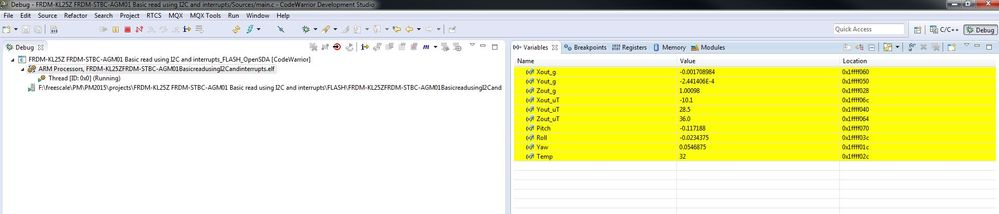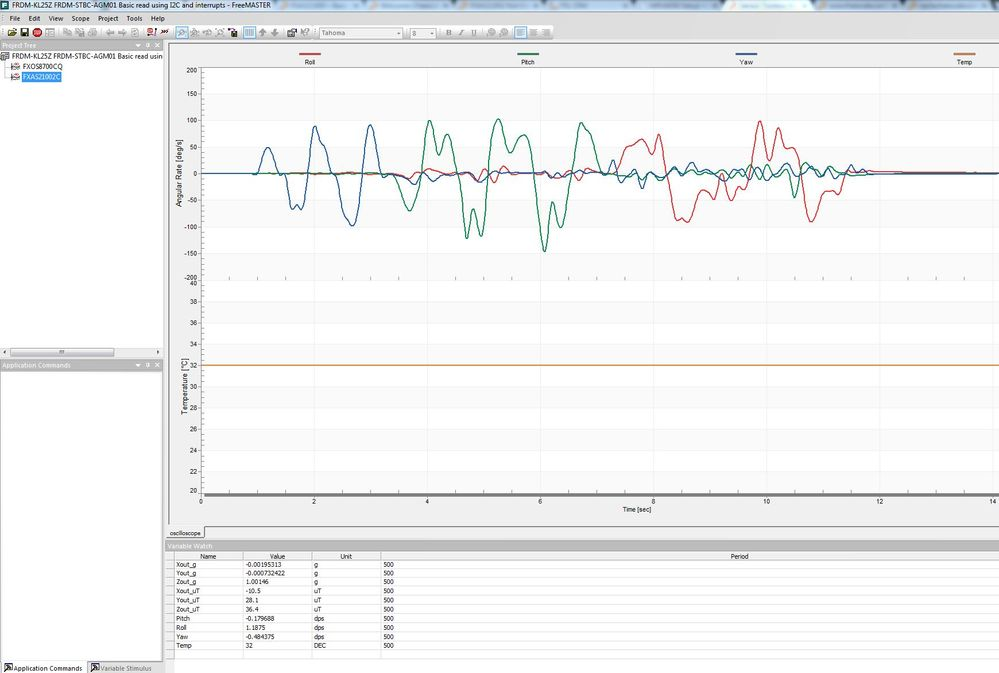I guess this is enough to let you start experimenting with the FRDM-STBC-AGM01 board. Attached you can find the complete source code written in the CW for MCU's v10.6 including the FreeMASTER project.

If there are any questions regarding this simple application, do not hesitate to ask below. Your feedback or suggestions are also welcome.

Regards,

Tomas

Labels (2)

• ### Magnetic Sensors

Version history
Revision #:
1 of 1
Last update:
‎07-22-2015 01:16 AM
Updated by: